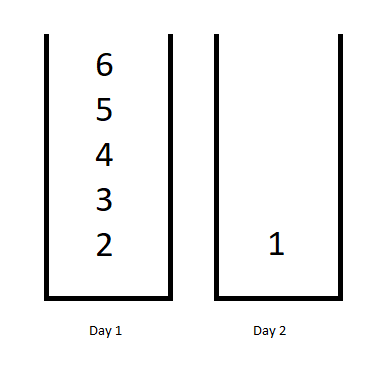# 工作计划的最低难度

## 1335. 工作计划的最低难度 (Hard)```输入：jobDifficulty = [6,5,4,3,2,1], d = 2

```

```输入：jobDifficulty = [9,9,9], d = 4

```

```输入：jobDifficulty = [1,1,1], d = 3

```

```输入：jobDifficulty = [7,1,7,1,7,1], d = 3

```

```输入：jobDifficulty = [11,111,22,222,33,333,44,444], d = 6

```

• `1 <= jobDifficulty.length <= 300`
• `0 <= jobDifficulty[i] <= 1000`
• `1 <= d <= 10`

[数组] [动态规划]